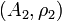# Tensor product of representations over a symmetric bimonoidal category

Suppose$G$ is a group and$\mathcal{C}$ is a symmetric bimonoidal category with operation$\oplus$ playing the role analogous to addition and operation$\otimes$ playing a role analogous to multiplication. Suppose$A_1,A_2$ are objects of$\mathcal{C}$ and$\rho_1:G \to \operatorname{Aut}(A_1)$ and$\rho_2:G \to \operatorname{Aut}(A_2)$ are group homomorphisms. We can thus think of$(A_1,\rho_1)$ and$(A_2,\rho_2)$ as representations of$G$ over$\mathcal{C}$. We define the tensor product of these representations, as a group homomorphism$\rho_1 \otimes \rho_2:G \to A_1 \otimes A_2$ where$(\rho_1 \otimes \rho_2)(g) = \rho_1(g) \otimes \rho_2(g)$ where the$\otimes$ on the right is the natural mapping$\operatorname{Aut}(A_1) \times \operatorname{Aut}(A_2) \to \operatorname{Aut}(A_1 \times A_2)$.
category of sets, where$\oplus$ is the coproduct (disjoint union) and$\otimes$ is the product (Cartesian product) tensor product of permutation representations
category of vector spaces over a field$K$ , where$\oplus$ is the direct sum of vector spaces (both the product and the coproduct) and$\otimes$ is the tensor product of vector spaces tensor product of linear representations